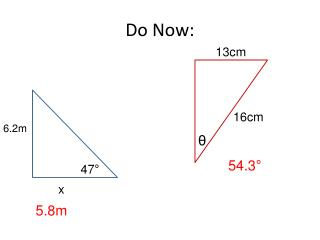DownloadDownload PresentationDo Now:

# Do Now:

Download Presentation## Do Now:

- - - - - - - - - - - - - - - - - - - - - - - - - - - E N D - - - - - - - - - - - - - - - - - - - - - - - - - - -
##### Presentation Transcript

1. Do Now: 13cm 16cm 6.2m θ 54.3° 47° x 5.8m

2. Rounding Decimals How many decimal places do each of these numbers have? 4.1 70 6.45 0.9873 0.007 456.88

3. Rounding Decimals When rounding decimals: • keep the required number of decimal places • if the next digit is < 5 (less than 5) keep the final required digit the same and drop all the unwanted digits off • if the next digit is ≥ 5 (greater than or equal to 5) round up by adding 1 to the final required digit and then dropping off all the unwanted digits.

4. Examples Round the following decimals to the number of decimal places indicated in the brackets a) 25.679 (1 dp) b) 0.0450 (3 dp) c) 56.2798 (2 dp) d) 1.04998 (3 dp) When solving a problem the answer should always be rounded to a sensible number of decimal places. The number of decimal places a number is rounded to should be written in brackets after the answer.

5. A 150cm plank of wood is divided into seven pieces. How long is each piece? 21.428 571cm It would be sensible to round this answer to 1dp or 2dp.

6. Recap of rounding decimals 4.88 • Round 4.875 to 2dp • Round 0.027942 to 2dp • Round 11.0498 to 3dp • A family uses 3578 litres of water in one week. How much water does the family use, on average, per day? (Round your answer to the nearest litre) 5) Here are the rainfall amounts for the five months of May, June, July, August and September. 59.3cm, 18.28cm, 25.91cm, 79.307cm and 18.4cm What is the total rainfall for this five-month period? 0.03 11.050 511L 201.2cm

7. Significant Figures • How many significant figures do these numbers have: 200 10300 1500 99 3.5 101 5.08 5 000 000 0.0073 0.04011

8. Zeros are significant when they are: • inbetween other numbers • Both at the end of a number and after the first non-zero digit after the decimal place. Examples: 9.703 (4sf) 27.40 (4sf) Zeros are not significant when they are: • At the front of a number • At the end of a number with no decimal point Examples: 0.036 (2sf) 24 000 (2sf) When rounding, using significant figures, it is often necessary to use zeros as place holders.

9. Examples • Round 389 to 1 significant figure • Round 2136 to 1 significant figure • Round 47 to 1 significant figure • Round 6451 to 2 significant figures

10. More examples.... • Round 0.04275 to 2 significant figures • Round 0.0035 to 1 significant figure • Round 0.060784 to 3 significant figures

11. 4000 Some for you to try: 3556 (1sf) 4368 (2sf) 417.3 (1sf) 0.00975 (2sf) 1843 (2sf) 654.9589 (4sf) 4400 400 0.0098 10Ticks worksheet L6 P3 pg 19 1800 655.0